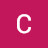Solve Equation Mentally 1 Tutor AlgebraSolve Equation Mentally 1 Tutor Algebra

This math video tutors and gives instruction about solving equations. The instruction is a brief and to the point teaching lesson on how to learn algebra and manipulate variables.
As you study Algebra, you will learn many techniques for solving equations. Some equations are simple enough to solve using mental math. Think of the equation as a question. Once you answer the question, check the solution.

algebra tutors,algebra,college,combine,educational,equation,expression,for,ged,help,high,homework,how to,instruction,learn,lesson,like terms,linear,math,mathematics,online,practice,pre,property,school,simplify,solve,solving,teach,tutor,tutorial,tutoring,variable,video,with

algebratutors.org

1.Chris27339 says: